# Switch Case Statements in C++

## Switch Case Statements in C++:

In this article, I am going to discuss Switch Case Statements in C++ with Examples. Please read our previous article where we discussed Dynamic Declaration in C++ with Examples.

##### Switch Case Statement in C++:

The ‘Switch’ is a branching and control statement. It is just like a switchboard at home whichever thing we want to put on we have to press that switch on.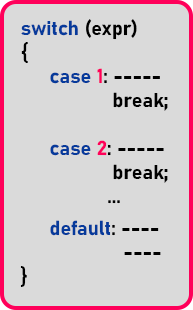This is the basic structure of the ‘switch-case’ statement. In the parenthesis of the switch, you can write some expression or a variable. But it can be either integer type, character type variable, or an expression. And for the different values of this expression, you can mention various cases.

In the above example, we have mentioned ‘case 1’, ‘case 2’ and after the keyword ‘case’ there is a space. Mostly, people do mistakes here. They don’t give space here. It will not be a syntax error but it will be a good programming practice to give space after the ‘case’ keyword.

Under the ‘case’ we need to break the case using the break statement, and here that case will end. The ‘default’ section is optional, if the cases are not mentioned or for the other cases, you can mention default.

##### How does Switch Case Statement work in C++?

Switch statement you can think like a switchboard where whatever you selected got executed instead of checking all the conditions. It means if you want to turn on the fan you need to switch on the fan switch directly similar way the switch works. To achieve the mechanism switch, make use of three keywords. Switch, case, and break;

Let us see how it works. Suppose the value of the given expression is ‘1’ then ‘case 1’ will be executed and stop when the break statement executes. And the switch statement break and control come out from the switch block.

If the value of the expression is ‘2’ then it will directly jump to the ‘case 2’ and stop at the break statement and control come out of the ‘switch’ statement.

If we mention the value of the expression as ‘9’ or apart from ‘1’ or ‘2’ then it will jump onto the default block and stop at break and the whole ‘switch’ statement will end there.

##### What happens if we don’t specify the break statement in the case block?

Suppose if we don’t give the break statement in ‘case 1’ then what happens? Now if we give the value of ‘1’ then this time both the cases will be executed as we didn’t have any break statement in ‘case 1’. So, if we don’t give a break statement in any case, then the next case will also be executed. So, for every case, you have to mention ‘break’. If you don’t need it then you can skip it.

The ‘default’ we can write it anywhere and it is optional. If it is written anywhere else then it should have ‘break’ followed by that. Now next thing if expression or variable is of ‘int’ type then cases will be ‘1’, ‘2’ and so on.

And if it is of ‘char’ type then we can use cases like ‘a’, ‘A’, ‘x’ and so on.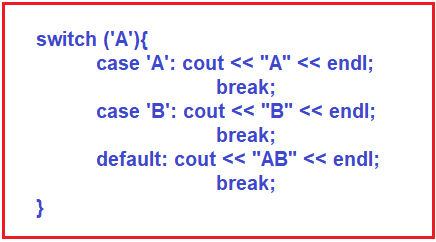So, we can even use character labels also.

##### Where this switch case is useful?

Mostly it is useful for writing menu-driven programs. For example, if I take the example of notepad, there are the options like ‘File’ and inside this file option ‘New’, ‘Open’, ‘Save’, ‘Save as’ etc. For each option, we can define some code i.e. ‘New – 1’, ‘Open – 2’, and so on. So, depending on the value we will define a particular case in the switch statement. One thing is that in any case, we can again define the switch statement. Below are some key points of the switch statement.

1. The switch is a branch and control statement
2. The switch can have 0 or more cases
3. Each case is defined with a label
4. Depending on the value of the expression in switch corresponding case black is executed
5. If a case block is not available then the default block is executed.
6. Default block is optional
7. Every case block must terminate with a break
8. If breaks are not mentioned then cases will fall through
9. The switch is used for menu-driven programs

Let us see some programs on the switch statement.

##### Program to Print Day Name using Switch Case in C++:
```#include<iostream>
using namespace std;

int main ()
{
int day;

cout << "Enter a day no. :" << endl;
cin >> day;

switch (day)
{
case 1:
cout << "Monday";
break;
case 2:
cout << "Tuesday";
break;
case 3:
cout << "Wednesday";
break;
case 4:
cout << "Thursday";
break;
case 5:
cout << "Friday";
break;
case 6:
cout << "Saturday";
break;
case 7:
cout << "Sunday";
break;
default:
cout << "Invalid day no." << endl;
}
return 0;
}
```
###### Output: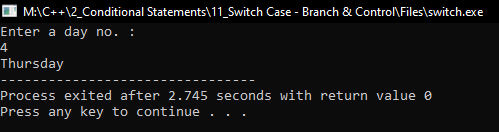##### Number Validation Program using Switch Case Statement in C++:
```#include<iostream>
using namespace std;

int main ()
{
int x = 1;

switch (x)
{
case 1:
cout << "One";
break;
case 2:
cout << "Two";
break;
case 3:
cout << "Three";
break;
default:
cout << "Invalid Number";
break;
}

return 0;
}
```
###### Output: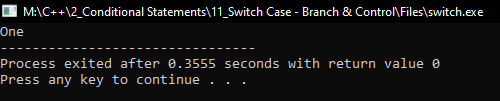##### Program to print Menu using Switch Case in C++:
```#include <iostream>
using namespace std;

int main ()
{
cout << "1. Add\n" << "2. Sub\n" << "3. Mul\n" << "4. Div\n";
int option;
cout << "Enter your choice no." << endl;
cin >> option;
int a, b, c;

cout << "Enter two numbers" << endl;
cin >> a >> b;

switch (option)
{
case 1:
c = a + b;
break;
case 2:
c = a - b;
break;
case 3:
c = a * b;
break;
case 4:
c = a / b;
break;
}

cout << c << endl;
return 0;
}
```
###### Output: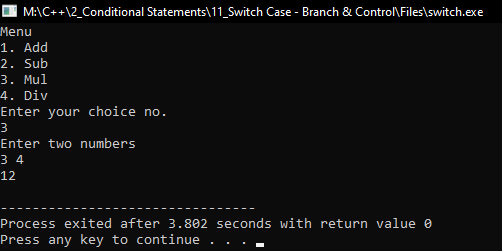##### Simple Calculator Program using Switch Case Statements in C++
```#include <iostream>
using namespace std;
int main ()
{
int a, b, result, choice;
cout << "enter the value of a and b\t" << endl;
cin >> a >> b;
cout <<"enter the choice press 1 for addition 2. for subtraction 3. for multiplication 4. for division" << endl;
cin >> choice;
switch (choice)
{
case 1:
result = a + b;
cout << "sum of two num is\t" << result << endl;
break;
case 2:
result = a - b;
cout << "difference of two num is\t" << result << endl;
break;
case 3:
result = a * b;
cout << "product of two num is\t" << result << endl;
break;
case 4:
result = a / b;
cout << "division of two num is\t" << result << endl;
break;
default:
cout << "you have entered a wrong choice" << endl;
}
}
```
###### Output: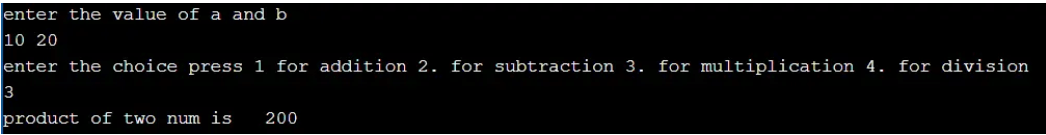In the next article, I am going to discuss Control Statement Programs in C++ with Examples. Here, in this article, I try to explain Switch Case Statements in C++ with Examples and I hope you enjoy this Switch Case Statements in C++ with Examples article.